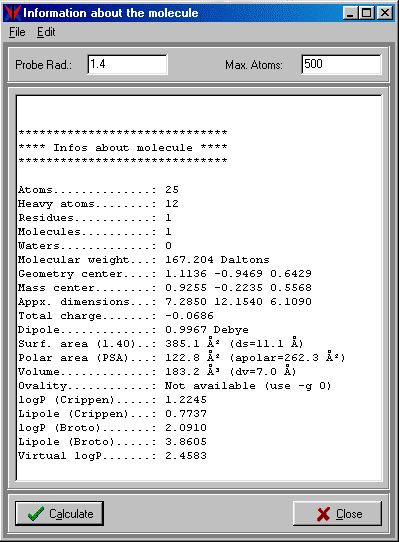3.3 Molecule AnalysisVEGA can calculate many information about the input molecule: total number of atoms, number of heavy atoms, number of residues, number of contained molecules, number of water molecules, molecular weight, coordinates of geometric center, coordinates of mass center, approximative dimensions, total charge (calculated using the atomic charges), dipole, surface area, surface diameter, volume, volume diameter, ovality, Crippen's logP (11) and lipole (12), Broto's logP (13) and lipole, Virtual logP(14), polypeptidic charge, aminoacidic or nucleotidic composition.VEGA can also evaluate the ligand-biomacromolecule interaction energy through the molecular mechanics calculation of non-bond term (R6-R12 Lennard-Jones) and coulombic term. To date, only the CVFF force field is implemented. For the energy evaluation, the user can specify the dielectric constant (default 1.0). After the energy calculation, VEGA shows (or writes in a file) the total interaction energy, its components (non-bond and coulombic energies) and a table with the receptor residues involved in interaction with a partial energy greater than 1% of the total energy. In this way it’s possible to improve the analysis of biomacromolecule - ligand complexes.